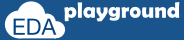# SystemC Functions

### Functions

function is group of statements to perform the specific task.

Syntax:

type function_name (arguments) {
function_body;
}

where,
type – functions will return an value, type specifies the return type
function_name – name of the function
arguments – arguments to the function
function_body – body of the function, which may contain declarations, assignments, expressions etc.
function can return a specific value by specifying return in function body, otherwise the result of last expression will be returned.

#### Example-1:

```#include "systemc.h"

{
return a+b;
}

int sc_main (int argc, char* argv[]) {
//declaration
int x,y,z;

//initialization
x=10;
y=20;

//function calling
cout <<" x+y = "<<z<<endl;

// Terminate simulation
return 0;
}
```

### void functions

Generally functions will return an value, where as void functions will not return any value.
by specifying type as void function can be declared as void function.

#### Example-1:

```#include "systemc.h"

void display (int a,int b)
{
cout <<" recived a = "<<a<<" b = "<<b<<endl;
}

int sc_main (int argc, char* argv[]) {

//function calling
display(10,20);

// Terminate simulation
return 0;
}
```

#### Simulator Output:

recived a = 10 b = 20
Execute the above code on### function call as expression

#### Example-1:

```#include "systemc.h"

{
return a+b;
}

int sc_main (int argc, char* argv[]) {
//declaration
int x,y,z;

//initialization
x=10;
y=20;

//function call in expression
z =  10 + add(x,y) + 20;
cout <<" Value of z = "<<z<<endl;

// Terminate simulation
return 0;
}
```

#### Simulator Output:

Value of z = 60
Execute the above code on### functions declaration

#### Example-1:

```#include "systemc.h"

//function display_1
void display_1 ()
{
display_2;
}

//function display_1
void display_2 ()
{
display_1;
}

int sc_main (int argc, char* argv[]) {

// Terminate simulation
return 0;
}
```

#### Simulator Output:

testbench.cpp: In function ‘void display_1()’:
testbench.cpp: error: ‘display_2’ was not declared in this scope
Exit code expected: 0, received: 1

The above example is locked situation where as display_1 is calling display_2 and display_2 is calling display_1, this leads to an compilation error.
This problem can be overcome by declaring the functions and writing the definitions separately.
Arguments  and type must match in declaration and definition.

#### Example-2:

```#include "systemc.h"

void display_1();
void display_2();

//function display_1
void display_1 ()
{
display_2();
}

//function display_1
void display_2 ()
{
display_1();
}

int sc_main (int argc, char* argv[]) {
cout <<"Inside Main"<<endl;
// Terminate simulation
return 0;
}
```